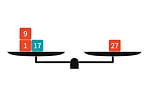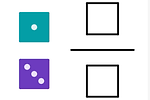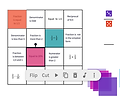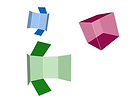top of page## Lesson Plans, Materials, Ideas, Activities

Here, you'll discover fun lessons, brain-teasing puzzles, and interesting activities to spark your curiosity about mathematics. Whether you're a student, teacher, or just someone curious about math, our content is here to make math enjoyable and accessible.

Dive right in and start your adventure in the world of math!

• Plane Figures

• Fractions

• Multiplication and Division with Multi-Digit Numbers

• Decimals

• Coordinate Plane

• Volume

• Rational Numbers, Exponents, LCM & GCF

• Ratio, Rate and Percentages

• Division Algorithms

• Decimals, Fractions, Fraction Division

• Expressions, Equations, and Inequalities

• Area, Surface Area, Coordinate Plane

• Data Sets and Distributions

• Rational Numbers

• Proportional Relationships, Scale Drawings, Percentages

• Circles

• Expressions, Equations, and Inequalities

• Angles, Triangles, and Prisms

• Probability and Sampling

• Rigid Transformations and Congruence

• Exponents and Scientific Notation

• Pythagorean Theorem and Irrational Numbers

• Dilations, Similarity

• Linear Relationships, and Equations, Systems of Eqıations, Slope

• Functions

• Volume

• Associations in Data# Fractions

##### Multiplying Fractions Using Modeling# Back to School

##### Balancing 1 to 40

Desmos Classroom Activity: Use the balance scale to balance all the numbers (weights) from 1-40 by using the numbers 1, 3, 9, and 27.# Fractions

##### Comparing Fractions

Desmos Classroom Activity:  Roll a pair of dice to create fractions that are less than 1/2, more than 1/2, and more than 1.# Decimals

##### Decimals: Multiplication and division with powers of 10

Desmos Classroom Activity: Using the place value chart to multiply and divide decimals with the powers of 10# Fractions

##### Fraction Bingo

Desmos Classroom Activity: Roll the dice and cover a square (or multiple squares) that describes your fraction. The winner is the first player to cover all the squares.# Decimals

##### Decimals Bingo

Desmos Classroom Activity: Use the spinners to multiply and divide decimals. Cover a square that describes the result of each operation. The winner is the first player to cover all the squares.# Volume

##### Missing Volume

Desmos Classroom Activity: Using unit cubes to determine the volume of a rectangular prism.# Volume

##### Hidden Cubes

Desmos Classroom Activity: Find the number of hidden cubes by creating the layered view of the given shapes.# Coordinate Plane

##### Plotting Points on the Coordinate Plane

Desmos Classroom Activity: Determine the ordered paırs for the given points and plot the points with the given coordinates.# Coordinate Plane

##### Lines on the Coordinate Plane

Desmos Classroom Activity: Draw lines by connecting the points with the given coordinates.# Plane  Figures

##### Definition(s) of Trapezoids

Desmos Classroom Activity: There are two different views regarding the definition of a trapezoid. Here are both views:# LCM & GCF

##### LCM & GCF Activity, Concept map and word problems

Polypad Activity, Desmos Classroom Activity, Worksheet# Prime Numbers

##### Divisibility and Prime Numbers# Expressions, Equations, and Inequalities

##### Tetromino Equations

Use algebraic expressions to write a general formula for the sum of the numbers covered by tetrominoes.# Area, Surface Area, Coordinate Plane

##### Surface Area and Volume of Rectangular Prisms

Fluency with Polypad: Fold and unfold prisms to calculate surface area and volume# Area, Surface Area, Coordinate Plane

##### Volume with Fractional Dimensions

Fluency with Polypad: Fold and unfold prisms to calculate their volume# Area, Surface Area, Coordinate Plane

##### Coordinate Plane

Play Star Wars Battleship Game with Polypad# Area, Surface Area, Coordinate Plane

##### Coordinate plane

Draw polygons in the coordinate plane given coordinates for the vertices; use coordinates to find the length of a side joining points and the area of the polygons.# Expressions, Equations

##### Tetromino Equations

Polypad Activity, Desmos Classroom Activity, Worksheet